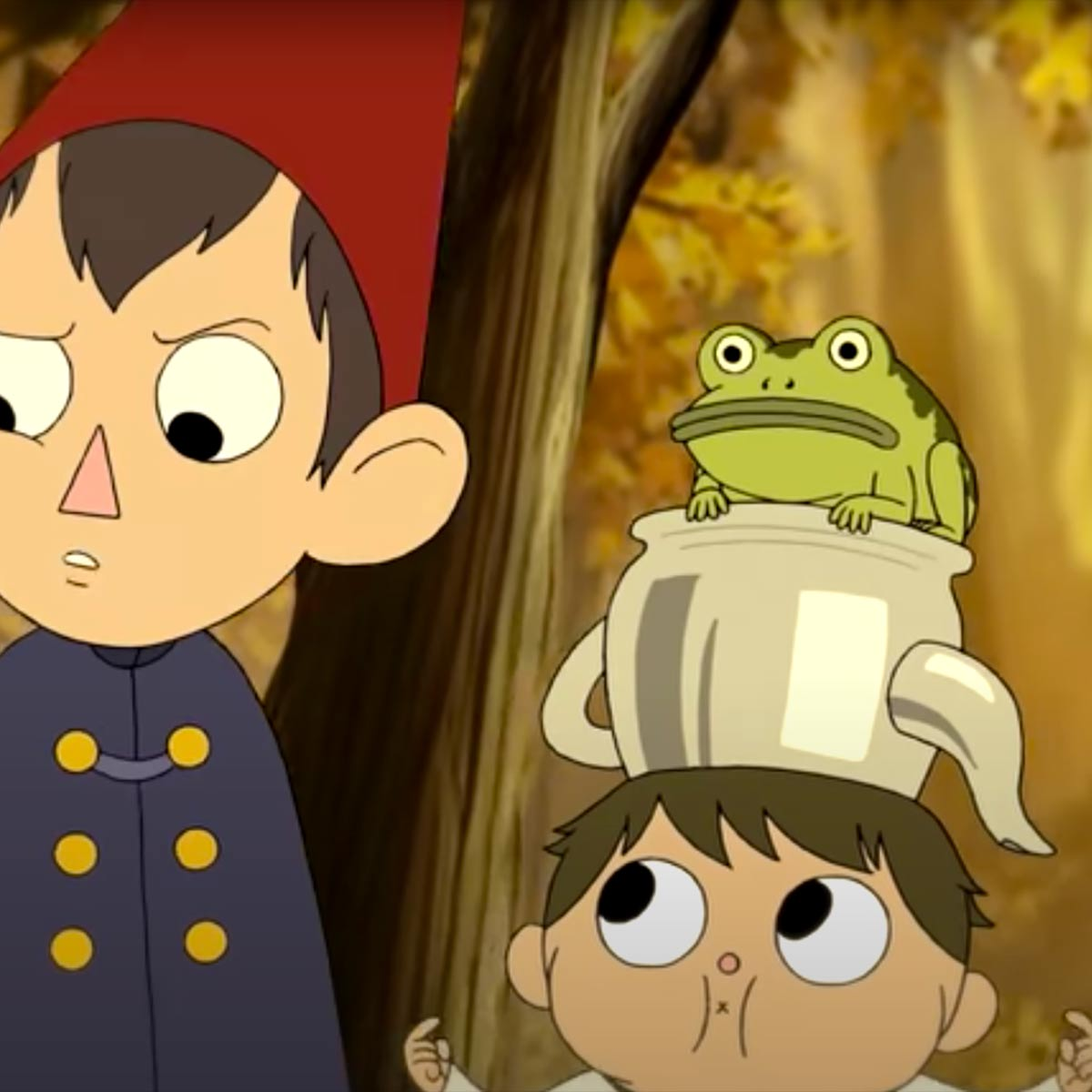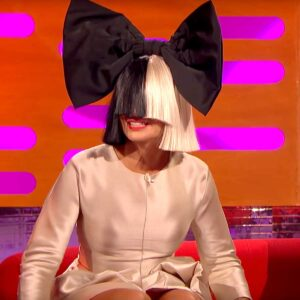# One is A Bird (Over the Garden Wall)

10 September 2020
2
• ##### TYPE
0
(0)

p a s d
s g fd
g D s d s asd
a s d f
d h gf
d k j hGh

Rate This Music Sheet:

Average rating 0 / 5. Vote count: 0

No votes so far! Be the first to rate this music sheet.

Thank you for rating this song!

If you have any specific feedback about how to improve this music sheet, please submit this in the box below.

One is A Bird (Over the Garden Wall) is a song by Blasting Company. Use your computer keyboard to play One is A Bird (Over the Garden Wall) music sheet on Virtual Piano. This is an Easy song and requires practice. The song One is A Bird (Over the Garden Wall) is classified in the genres: Classical, Songs From TV on Virtual Piano. You can also find other similar songs using Anime.

## Related

•[VQ] 9 Gfe pfO *aSapI \$ SSS SSS SSS 9dd dddfda 6 aSS aSS aSfS *aSap[IQ] SS SSS SSS 9dd dd ddd fd 6 aSS aaaSfS

Level: 3
Length: 00:40
Easy

#### Sia

•f f f f d f j f dd | d d d d s d h d s a s | pas s s s s | pas s s s s a s | pas s s s s h | s d | f f f f d f j f dd | d d d d s d h d s a s | pas s s s s | pas s s s s a s | pas s s s s h | g D d | f | g | h h | h h g f d d fs | fg h h | h h g f d d f | s a f | p s | s d f | p s | j k l l z l k f h j j h g h o o o i i Y i t i o | i o j k l l z l k f h j j h g h | h h g g D g h H J H H h H h H J l | H J l | l k l z | h l k l | f f d f j f j j j j h j z k j l | k | h | j | v | c | l | z | | f d f k f | d s d h d | pas | p a s d f | j k l l z l k k f h j j h g h h o o i i Y i | t i o | O o p a s s d s a u o p p o i o o o i i Y i o O P O O o O o O P s O P s | s a s d | o s a s
Level: 3
Length: 02:29
Easy

#### Joe Hisaishi

•u p s [tp] p s p [ya] p i o [ue] |
u p s [tp] p s p [ew] p u Y [yw] |
y i O [rw] |
y i O [pw] |
e t o [iw] u o i [iw] u u W [ew]

Level: 4
Length: 00:30
Easy